# Learning Mathematics Effectively Through A Complete Quiz

This quiz contains lots of different questions of mathematics including various topics like number theory, decimals, integers, fractions, measurement, probability and so on, covering the course content of mathematics of Grade 7. Various types of questions are included in the quiz to make it more interactive and many real-life examples are used to let students feel the importance of mathematics.

Start Quiz

What is the Least Common Multiple of 4 and 12?

##12##

12

Type the missing integer.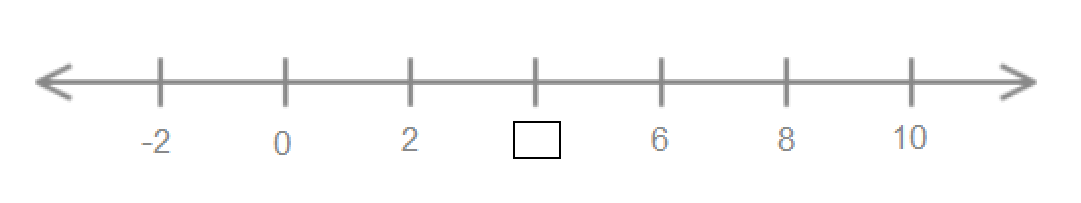##4##

4

Subtract the given integers.

12 - (-1) = ##13##

13

Subtract the given decimals.

763.37 - 93.70= ##669.67##

669.67

A chocolate bar is 3/4 of an inch long. If it is divided into pieces that are 3/8 of an inch long, then how many pieces is that?

2

3

4

5

Is √2 a rational number?

yes

no

William’s pencil box contains 3 pencils and 2 erasers. What is the ratio of erasers to pencils in William’s pencil box?

3:2

2:3

15 flowers in 5 vases = ##3## flowers per vase

3

What percent of the given figure is yellow?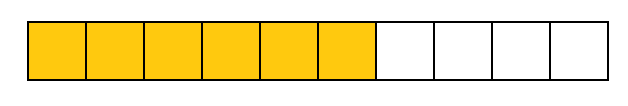##60## percent

60

There are 50 students in a class. If 14% are absent on a particular day, find the number of students absent in the class.

12

7

16

14

Sam sold 1 cookie on the 1st day, 3 cookies on the 2nd day, 5 cookies on the 3rd day, 7 cookies on the 4th day, and so on. How many cookies would Sam eat on the 10th day?

19

What value of c is a solution to this equation?

2c + 1 =5

c= ##2##

2

Are the given triangles congruent?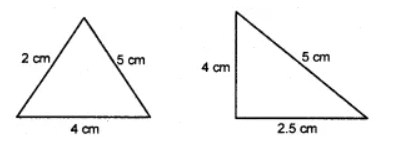yes

no

If the given triangles are congruent, find the length of LN.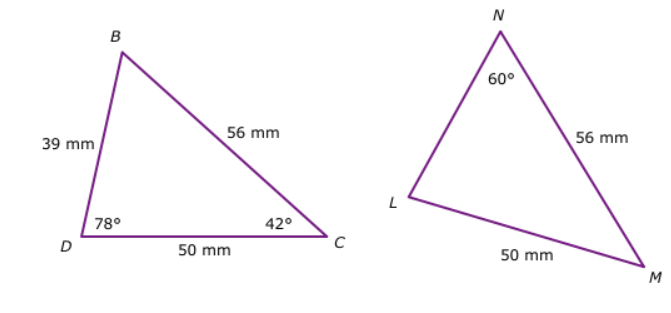LN = ##39## millimeters

39

The lines PR is parallel to SU are parallel to each other.

Choose the correct pair of interior alternate angles.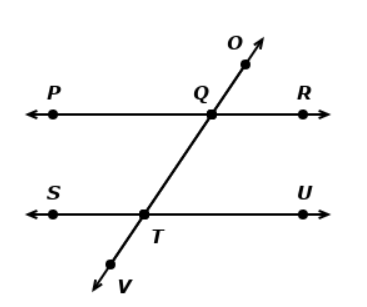Angle PQO and Angle OQR

Angle QTU and Angle QTS

Angle STQ and Angle PQT

Angle RQT and Angle UTQ

A rectangular schoolyard is 150 meters long and 200 meters wide. Find the perimeter of the schoolyard.

Perimeter= ##700## meters

700

A card is drawn from a pack of 52 cards. Find the probability that it is neither a king nor a jack.

11/26

11/13

11/14

21/52

A glass jar contains 5 red, 7 green, 9 blue, and 11 yellow marbles. If a single marble is picked at random from the jar, what is the probability of it being a blue marble?

1/4

3/17

21/32

9/32

What is the probability of drawing an ace card from a pack of 52 cards?

1/13

4/13

12/52

9/52

A recipe requires 1/4 teaspoon cayenne pepper, 3/4 teaspoon black pepper, and 1/4 teaspoon red pepper. How much pepper does this recipe need?

2/3

5/2

5/4

7/4

Quiz/Test Summary
Title: Learning Mathematics Effectively Through A Complete Quiz
Questions: 20
Contributed by: